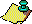Contents Next: The Miracle of Up: RamanujanModular Equations, Previous: iii) Transformation Methods

# Complexity Concerns

One of the interesting morals from theoretical computer science is that many familiar algorithms are far from optimal. In order to be more precise we introduce the notion of bit complexity. Bit complexity counts the number of single operations required to complete an algorithm. The single-digit operations are +, -,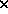. (We could, if we wished, introduce storage and logical comparison into the count. This, however, doesn't affect the order of growth of the algorithms in which we are interested.) This is a good measure of time on a serial machine. Thus, addition of two n-digit integers by the usual method has bit complexity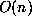, straightforward uniqueness considerations show this to be asymptotically best possible.

Multiplication is a different story. Usual multiplication of two n-digit integers has bit complexity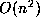and no better. However, it is possible to multiply two n-digit integers with complexity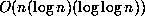. This result is due to Schönhage and Strassen and dates from 1971 . It provides the best bound known for multiplication. No multiplication can have speed better than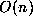. Unhappily, more exact results aren't available.

The original observation that a faster than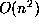multiplication is possible was due to Karatsuba in 1962. Observe that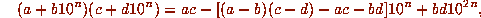and thus multiplication of two 2n-digit integers can be reduced to three multiplications of n-digit integers and a few extra additions. (Of course multiplication by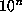is just a shift of the decimal point.) If one now proceeds recursively one produces a multiplication with bit complexity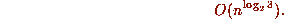Note that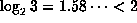. We denote by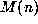the bit complexity of multiplying two n-digit integers together by any method that is at least as fast as usual multiplication.

The trick to implementing high precision arithmetic is to get the multiplication right. Division and root extraction piggyback off multiplication using Newton's method. One may use the iteration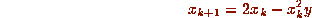to compute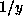and the iteration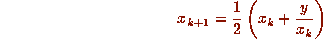to compute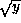. One may also compute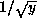from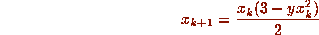and so avoid divisions in the computation of. Not only do these iterations converge quadratically but, because Newton's method is self-correcting (a slight perturbation in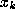does not change the limit), it is possible at the kth stage to work only to precision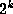. If division and root extraction are so implemented, they both have bit complexity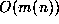, in the sense that n-digit input produces n-digit accuracy in a time bounded by a constant times the speed of multiplication. This extends in the obvious way to the solution of any algebraic equation, with the startling conclusion that every algebraic number can be computed (to n-digit accuracy) with bit complexity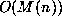. Writing down n digits of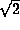oris (up to a constant) no more complicated than multiplication. The Schönhage-Strassen multiplication is hard to implement. However, a multiplication with complexity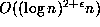based on an ordinary complex (floating point) fast Fourier transform is reasonably straightforward. This is Kanada's approach, and the recent records all rely critically on some variations of this technique.

To see how the fast Fourier transform may be used to accelerate multiplication, let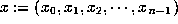and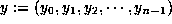be the representations of two high-precision numbers in some radix b. The radix b is usually selected to be some power of 2 or 10 whose square is less than the largest integer exactly representable as an ordinary floating-point number on the computer being used. Then, except for releasing each ``carry'', the product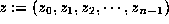of x and y may be written as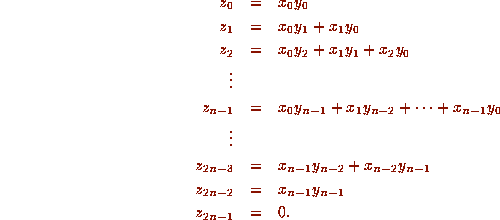Now consider x and y to have n zeros appended, so that x, y, and z all have length N =2n. Then a key observation may be made: the product sequence z is precisely the discrete convolution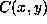: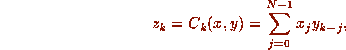where the subscript k-j is to be interpreted as k-j +N if k-j is negative.

Now a well-known result of Fourier analysis may be applied. Let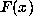denote the discrete Fourier transform of the sequence x, and let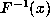denote the inverse discrete Fourier transform of x: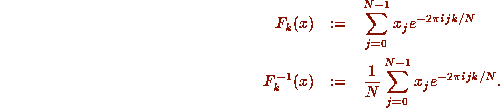Then the ``convolution theorem'', whose proof is a straightforward exercise, states that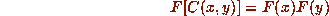or, expressed another way,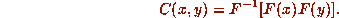Thus the entire multiplication pyramid z can be obtained by performing two forward discrete Fourier transforms, one vector complex multiplication and one inverse transform, each of length N =2n. Once the real parts of the resulting complex numbers have been rounded to the nearest integer, the final mutiprecision product may be obtained by merely releasing the carries modulo b. This may be done by starting at the end of the z vector and working backward, as in elementary school arithmetic, or by applying other schemes suitable for vector processing on more sophisticated computers.

A straightforward implementation of the above procedure would not result in any computational savings --- in fact, it would be several times more costly than the usual ``schoolperson'' scheme. The reason this scheme is used is that the discrete Fourier transform may be computed much more rapidly using some variation of the well-known ``fast Fourier transform'' (FFT) algorithm . In particular, if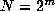, then the discrete Fourier transform may be evaluated in only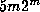arithmetic operations using an FFT. Direct application of the definition of the discrete Fourier transform would require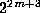floating-point arithmetic operations, even if it is assumed that all powers of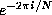have been precalculated.

This is the basic scheme for high-speed multiprecision multiplication. Many details of efficient implementations have been omitted. For example, it is possible to take advantage of the fact that the input sequences x and y and the output sequence z are all purely real numbers, and thereby sharply reduce the operation count. Also, it is possible to dispense with complex numbers altogether in favor of performing computations in fields of integers modulo large prime numbers. Interested readers are referred to , , , and . When the costs of all the constituent operations, using the best known techniques, are totalled both Algorithms 1 and 2 compute n digits ofwith bit complexity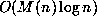, and use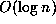full precision operations.

The bit complexity for Sum 1, or forusing any of the arctan expansions, is between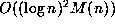and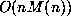depending on implementation. In each case, one is required to sumterms of the appropriate series. Done naively, one obtains the latter bound. If the calculation is carefully orchestrated so that the terms are grouped to grow evenly in size (as rational numbers) then one can achieve the former bound, but with no corresponding reduction in the number of operations. The Archimedean iteration of section 2 converges like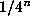so in excess of n iterations are needed for n-digit accuracy, and the bit complexity is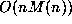.

Almost any familiar transcendental number such as e,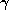,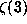, or Catalan's constant (presuming the last three to be nonalgebraic) can be computed with bit complexity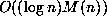or. None of these numbers is known to be computable essentially any faster than this. In light of the previous observation that algebraic numbers are all computable with bit complexity, a proof thatcannot be computed with this speed would imply the transcendence of. It would, in fact, imply more, as there are transcendental numbers which have complexity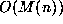. An example is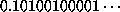. It is also reasonable to speculate that computing the nth digit ofis not very much easier than computing all the first n digits. We think it very probable that computing the nth digit ofcannot be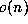.Contents Next: The Miracle of Up: RamanujanModular Equations, Previous: iii) Transformation Methods